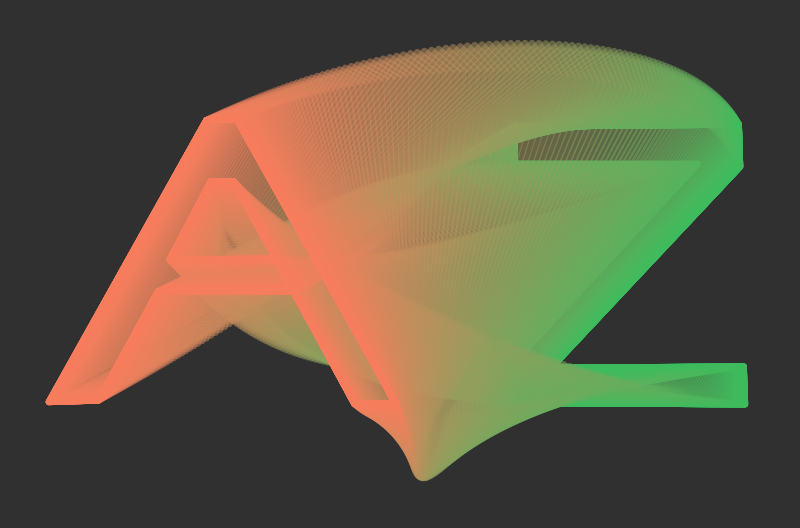# Clayton ShonkwilerThe paper “Stiefel manifolds and polygons” describes an interpretation of polygons as points in certain Stiefel manifolds, and gives some examples of how to use this interpretation for both generative and deterministic purposes.

This correspondence is based on our work in the paper “Probability theory of random polygons from the quaternionic viewpoint”, with Jason Cantarella and Tetsuo Deguchi; see also “Random triangles and polygons in the plane”; this work is devoted more to the aesthetic possibilities of this correspondence.

The above animation shows a geodesic in the Grassmannian $$\operatorname{Gr}_2(\mathbb{C}^{200})$$ between the blackboard-framed regular 200-gon and the regular 200-gon with a thrice-twisted frame. And here is a path in the Stiefel manifold $$\operatorname{St}_2(\mathbb{R}^{12})$$:The figures in this paper were produced using this Mathematica notebook, which unfortunately requires access to Mathematica to open. However, it is also available in Wolfram CDF format (which can be opened using the free Wolfram CDF Player) or as a static PDF.

Warning: All of the above are large files (∼50 MB for the notebook and CDF, ∼6MB for the PDF).